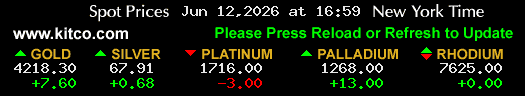Enter Weight

Grams (g)

Troy Oz. Pure Gold

0.0000

Gross Melt Value

\$ 0.00

Profit \ Loss

+\- %

P\L Value

\$ 0.00

Note: The grid view feaure is only available for Pro members.

### Gold Valuation Tools:

1. Gold Testing Kit. Want to know if your gold is real? A simple acid test will give you confirmation.

2. Digital Scale. When weighing gold you want to be as precise as possible. We recommend a scale that is accurate to .01 or 1/100 gram.

USD

#### 10 Karat

 1 gram = 21.20 1 .dwt = 32.97 5 grams = 105.99 20 grams = 423.98 1 troy oz. = 659.36

#### 14 Karat

 1 gram =29.64 1 .dwt =46.09 5 grams =148.19 20 grams =592.76 1 troy .oz =921.84

#### 18 Karat

 1 gram =38.13 1 .dwt =59.30 5 grams =190.64 20 grams =762.55 1 troy .oz =1,185.90

#### 22 Karat

 1 gram =46.62 1 .dwt =72.50 5 grams =233.09 20 grams =932.35 1 troy .oz =1,449.96

USD

1 gram

50.84

2.5 grams

127.09

.10 troy .oz

158.12

5 grams

254.18

.25 troy .oz

395.30

10 grams

508.37

.5 troy .oz

790.60

20 grams

1,016.74

50 grams

2,541.84

100 grams

5,083.68